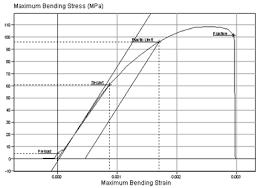## How to Calculate and Solve for Flexural Strength for Circular Cross-section in Defects | CeramicsThe image above represents flexural strength for circular cross-section in defects.

To compute for flexural strength for circular cross-section in defects, three essential parameters are needed and these parameters are Load at fracture (Ff), Specimen radius (R) and Distance between support Points (L).

The formula for calculating flexural strength for circular cross-section in defects:

σfs = FfL / πR³

Where:

σfs = Flexural Strength
L = Distance between Support Points

Lets’s solve an example;
Find the flexural strength when the distance between support points is 30, load at fracture is 21 and the specimen radius is 11.

This implies that;

L = Distance between Support Points = 30
Ff = Load at Fracture = 21
R = Specimen Radius = 11

σfs = FfL / πR³
σfs = (21)(30) / π(11)³
σfs = (630) / π(1331)
σfs = (630) / (4181.4)
σfs = 0.150

Therefore, the flexural strength for circular cross-section is 0.150 Pa.

Calculating the Distance Between Support Points when the Flexural Strength for Circular Cross-section, the Specimen Radius and the Load at Fracture is Given.

L = σfs x πR³ / Ff

Where;

L = Distance between Support Points
σfs = Flexural Strength

Let’s solve an example;
Find the distance between support points when the flexural strength is 44, load at fracture is 3 and specimen radius is 20.

This implies that;

σfs = Flexural Strength = 44
Ff = Load at Fracture = 3
R = Specimen Radius = 20

L = σfs x πR³ / Ff
L = 44 x π x 3³ / 20
L = 44 x π x 27 / 20
L = 3732.2 / 20
L = 186.61

Therefore, the distance between support point is 186.61.